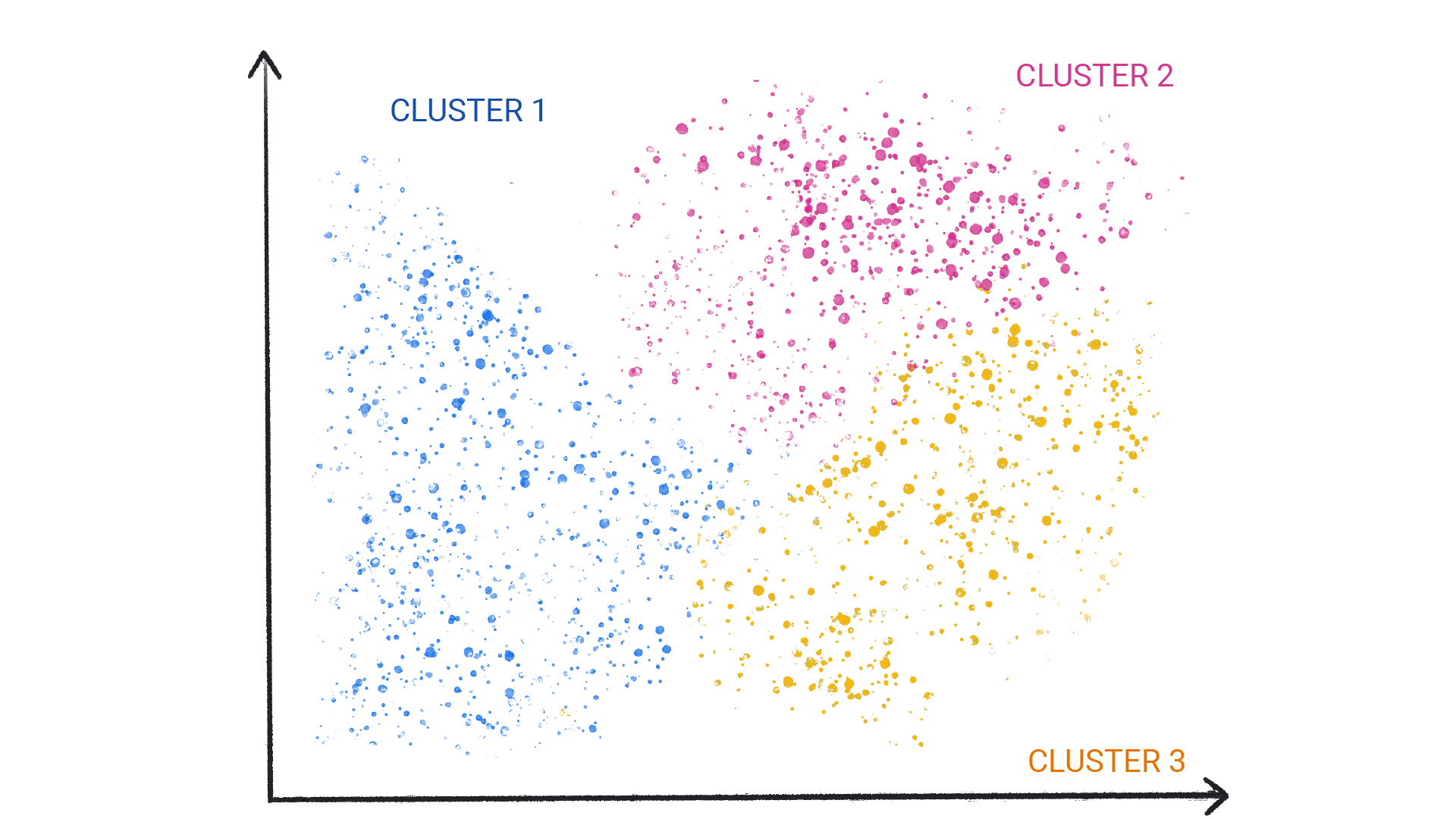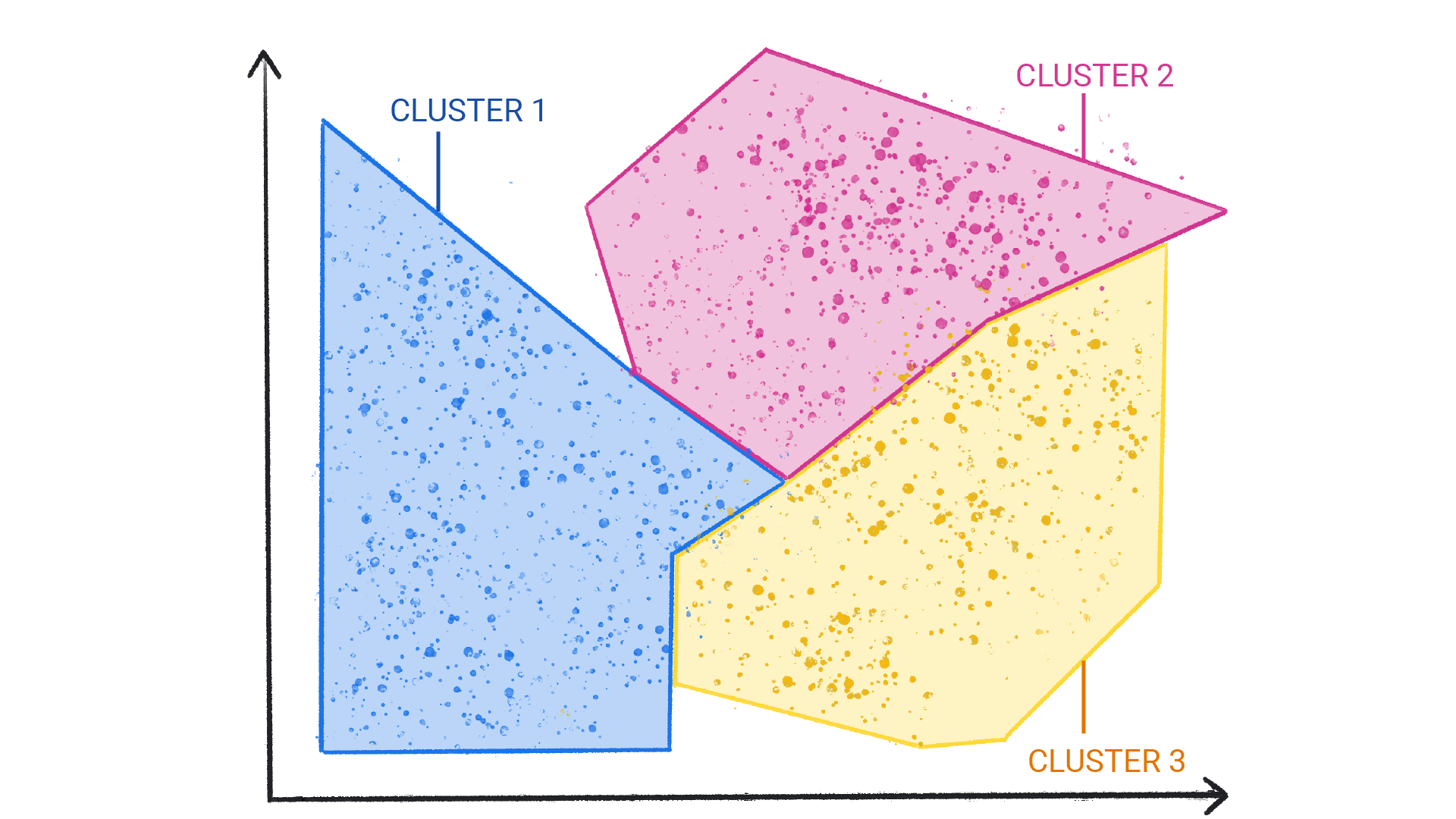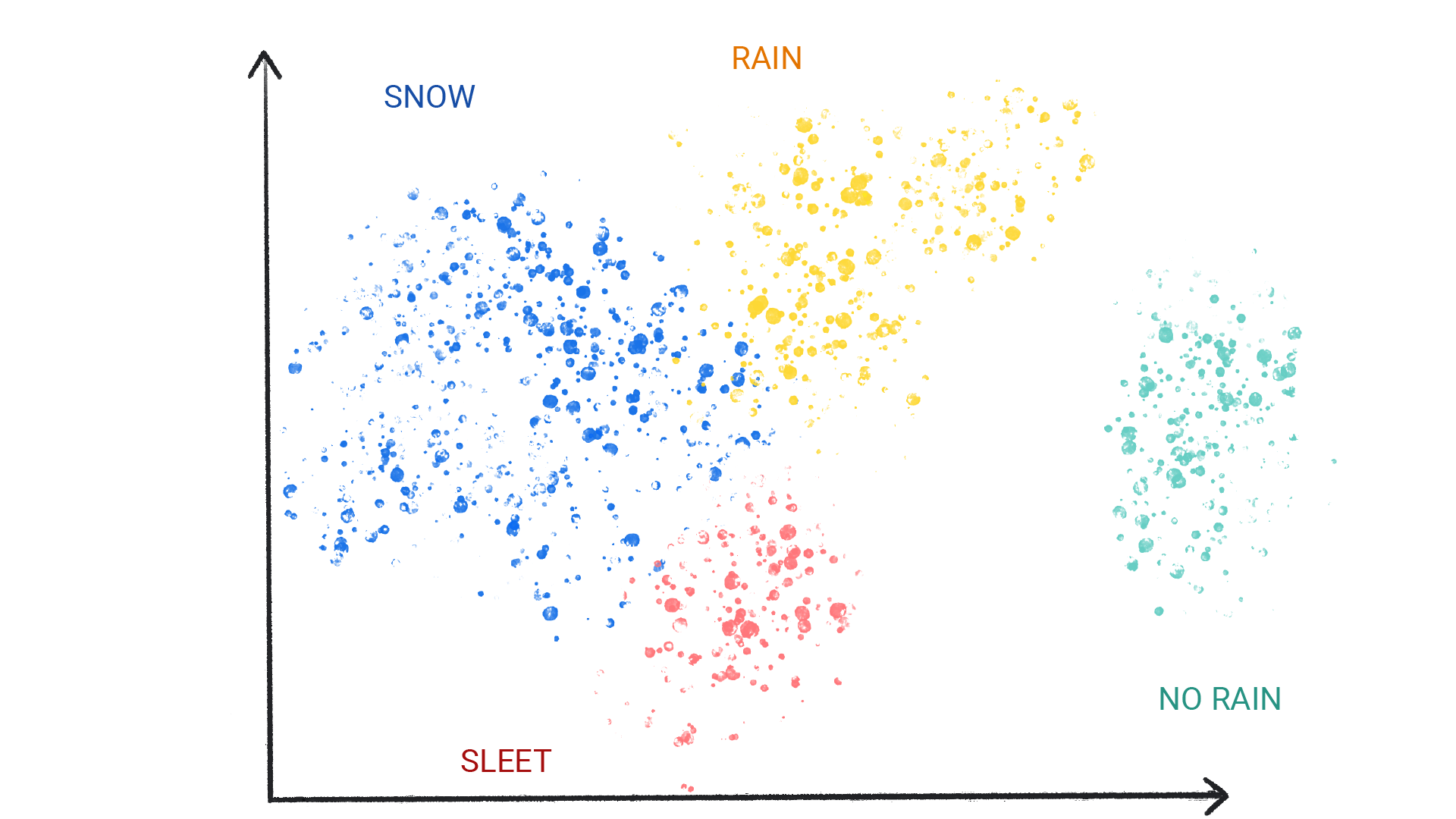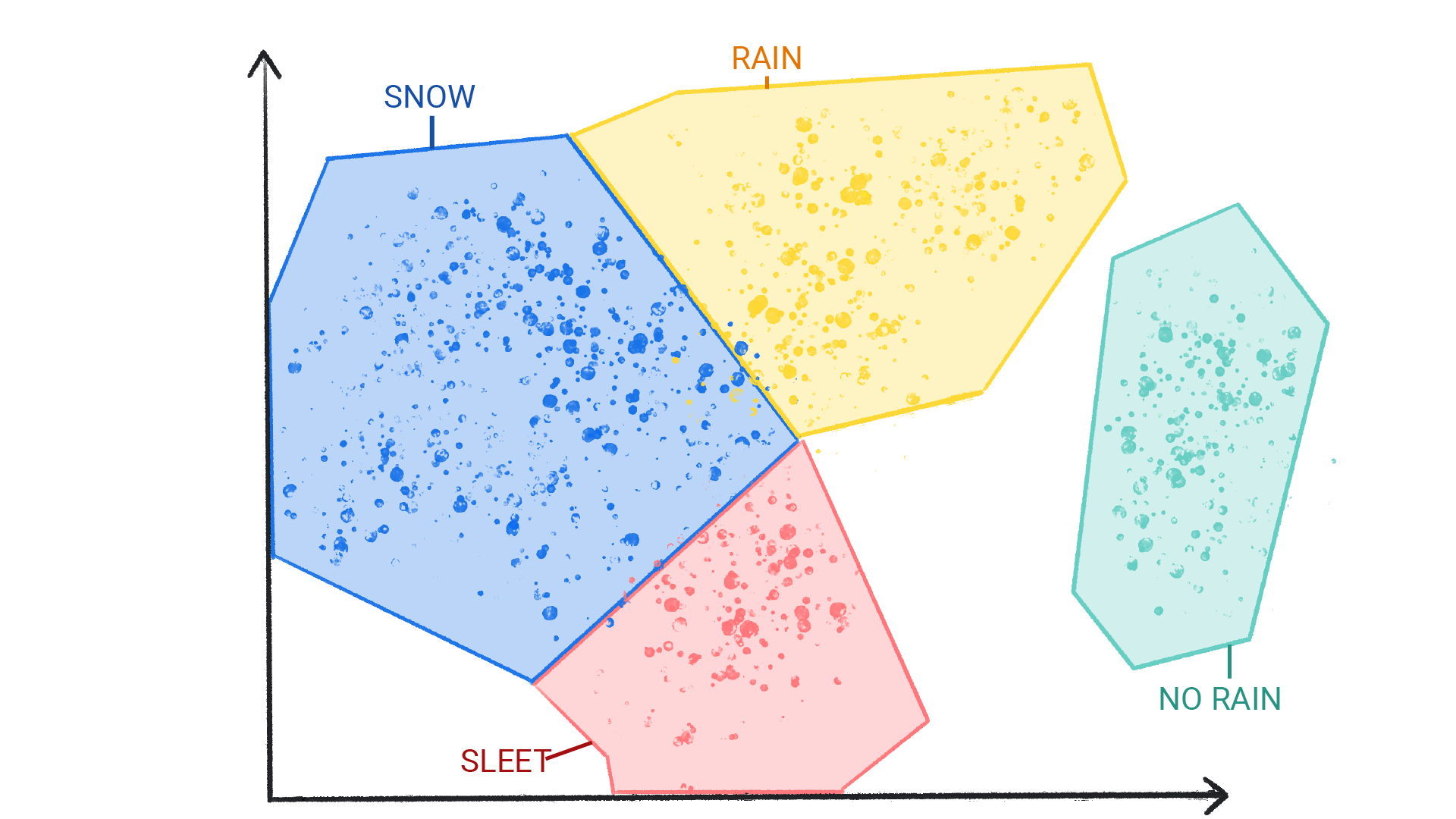# What is Machine Learning?

Machine learning (ML) powers some of the most important technologies we use, from translation apps to autonomous vehicles. This course explains the core concepts behind ML.

ML offers a new way to solve problems and answer complex questions. In basic terms, ML is the process of training a piece of software, called a model, to make useful predictions from data. An ML model represents the mathematical relationship between the elements of data that an ML system uses to make predictions.

For example, suppose we wanted to create an app to predict rainfall. We could use either a traditional approach or an ML approach. Using a traditional approach, we'd create a physics-based representation of the Earth’s atmosphere and surface, computing massive amounts of fluid dynamics equations. This is incredibly difficult.

Using an ML approach, we would give an ML model enormous amounts of weather data until the ML model eventually learned the mathematical relationship between weather patterns that produce differing amounts of rain. We would then give the model the current weather data, and it would predict the amount of rain.

What is a "model” in machine learning?
A model is a mathematical relationship derived from data that an ML system uses to make predictions
A model is a piece of computer hardware
A model is a smaller representation of the thing you're studying.

## Types of ML Systems

ML systems fall into three distinct categories based on how they learn to make predictions:

• Supervised learning
• Unsupervised learning
• Reinforcement learning

## Supervised learning

Supervised learning models can make predictions after seeing lots of data with the correct answers and then discovering the connections between the elements in the data that produce the correct answers. This is like a student learning new material by studying old exams that contain both questions and answers. Once the student has trained on enough old exams, the student is well prepared to take a new exam. These ML systems are “supervised” in the sense that a human gives the ML system data with the known correct results.

Two of the most common use cases for supervised learning are regression and classification.

### Regression

A regression model predicts a numeric value. For example, a weather model that predicts the amount of rain, in inches or millimeters, is a regression model.

See the table below for more examples of regression models:

Scenario Possible input data Numeric prediction
Future house price Square footage, zip code, number of bedrooms and bathrooms, lot size, mortgage interest rate, property tax rate, construction costs, and number of homes for sale in the area. The price of the home.
Future ride time Historical traffic conditions (gathered from smartphones, traffic sensors, ride-hailing and other navigation applications), distance from destination, and weather conditions. The time in minutes and seconds to arrive at a destination.

### Classification

Classification models predict the likelihood that something belongs to a category. Unlike regression models, whose output is a number, classification models output a value that states whether or not something belongs to a particular category. For example, classification models are used to predict if an email is spam or if a photo contains a cat.

Classification models are divided into two groups: binary classification and multiclass classification. Binary classification models output a value from a class that contains only two values, for example, a model that outputs either rain or no rain. Multiclass classification models output a value from a class that contains more than two values, for example, a model that can output either rain, hail, snow, or sleet.

If you wanted to use an ML model to predict energy usage for commercial buildings, what type of model would you use?
Regression
Energy usage is measured in kilowatthours (kWh), which is a number, so you'd want to use a regression model.
Classification
Classification models predict whether or not something belongs to a category, while regression models predict a number. Because energy usage is measured in kilowatthours (kWh), which is a number, you'd want to use a regression model.

## Unsupervised learning

Unsupervised learning models make predictions by being given data that does not contain any correct answers. An unsupervised learning model's goal is to identify meaningful patterns among the data. In other words, the model has no hints on how to categorize each piece of data, but instead it must infer its own rules.

A commonly used unsupervised learning model employs a technique called clustering. The model finds data points that demarcate natural groupings.Figure 1. An ML model clustering similar data points.Figure 2. Groups of clusters with natural demarcations.

Clustering differs from classification because the categories aren't defined by you. For example, an unsupervised model might cluster a weather dataset based on temperature, revealing segmentations that define the seasons. You might then attempt to name those clusters based on your understanding of the dataset.Figure 3. An ML model clustering similar weather patterns.Figure 4. Clusters of weather patterns labeled as snow, sleet, rain, and no rain.

What distinguishes a supervised approach from an unsupervised approach?
A supervised approach is given data that contains the correct answer.
A supervised approach is given data that contains the correct answer. The model's job is to find connections in the data that produce the correct answer. An unsupervised approach is given data without the correct answer. Its job is to find groupings in the data.
A supervised approach typically uses clustering.
An unsupervised approach uses clustering.
An unsupervised approach knows how to label clusters of data.
An unsupervised approach doesn't know what the clusters of data mean. Based on your understanding of the data, it's up to you to define them.

## Reinforcement learning

Reinforcement learning models make predictions by getting rewards or penalties based on actions performed within an environment. A reinforcement learning system generates a policy that defines the best strategy for getting the most rewards.

Reinforcement learning is used to train robots to perform tasks, like walking around a room, and software programs like AlphaGo to play the game of Go.

[{ "type": "thumb-down", "id": "missingTheInformationINeed", "label":"Missing the information I need" },{ "type": "thumb-down", "id": "tooComplicatedTooManySteps", "label":"Too complicated / too many steps" },{ "type": "thumb-down", "id": "outOfDate", "label":"Out of date" },{ "type": "thumb-down", "id": "samplesCodeIssue", "label":"Samples / code issue" },{ "type": "thumb-down", "id": "otherDown", "label":"Other" }]
[{ "type": "thumb-up", "id": "easyToUnderstand", "label":"Easy to understand" },{ "type": "thumb-up", "id": "solvedMyProblem", "label":"Solved my problem" },{ "type": "thumb-up", "id": "otherUp", "label":"Other" }]